Courses

# DC Pandey Solutions: Current Electricity- 2 Notes | EduRev

## JEE : DC Pandey Solutions: Current Electricity- 2 Notes | EduRev

The document DC Pandey Solutions: Current Electricity- 2 Notes | EduRev is a part of the JEE Course DC Pandey Solutions for NEET Physics.
All you need of JEE at this link: JEE

Introductory Exercise 20.4

Ques 1: In the circuit shown in figure, a 12 V power supply with unknown internal resistance r is connected to a battery with unknown emf. E and internal resistance 1Ω  and to a resistance of 3Ω . carrying a current of 2 A. The current through the rechargeable battery is 1 A in the direction shown. Find the unknown current i, internal resistance r and the emf E.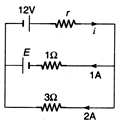Ans:
Applying loop law equation in upper loop we have,
E + 12 - ir - 1 = 0 ...(i)
Applying loop law equation in lower loop we have where i = 1 + 2 = 3A
E + 6 - 1 = 0 ...(ii)
Solving these two equations we get, E = -5Vand r = 2Ω

Ques 2: In the above example, find the power delivered by the 12 V power supply and the power dissipated in 3 W resistor.
Ans:
Power delivered by a battery = Ei
= 12 × 3 = 36 W
Power dissipated in resistance = i2R
= (3) (2)2 = 12W

Introductory Exercise 20.5

Ques 1: Find the equivalent emf and internal resistance of the arrangement shown in Fig.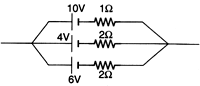Ans: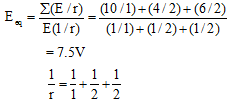r = 0.5 W

Ques 2: If a battery of emf E and internal resistance r is connected across a load of resistance R. Show that the rate at which energy is dissipated in R is maximum when R = r and this maximum power is P = E2/4r.
Ans: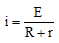P = power across R = i2R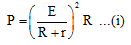For power to be maximum,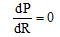By putting weget, R = r
Further, by putting R = r in Eq. (i)
we get,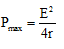Ques 3: Two identical batteries each of emf E = 2 volt and internal resistance r =1 ohm are available to produce heat in an external resistance by passing a current through it. What is the maximum power that can be developed across an external resistance R using these batteries.
Ans:
As derived in the above question,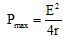Here, E = net emf = 2 + 2 = 4 V
and r= net internal resistance
= 1 + 1 = 2Ω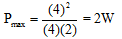Ques 4: The full scale deflection current of a galvanometer of resistance 1Ω is 5 mA. How will you convert it into a voltmeter of range 5 V ?
Ans:
V = ig(G + R)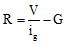= series resistance connected with galvanometer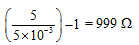Ques 5: A micrometer has a resistance of 100Ω and full scale deflection current of 50μA. How can it be made to work as an ammeter of range 5 mA ?
Ans: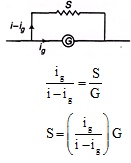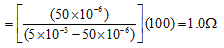Ques 6: A voltmeter has a resistance G and range V. Calculate the resistance to be used in series with it to extend its range to nV.
Ans:
V = igG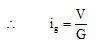Now, nV = ig(G + R) = V/G (G + R) R = (n - 1)G

Ques 7: The potentiometer wire AB is 600 cm long.
(a) At what distance from A should the jockey J touch the wire to get zero deflection in the galvanometer.
(b) If the jockey touches the wire at a distance 560 cm from A, what will be the current through the galvanometer.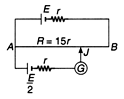Ans:
(a)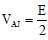or emf of lower battery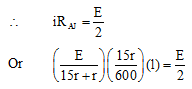Solving this equation we get,
l = 320 cm

(b) Resistance of 560 cm =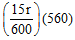= 14r Now the circuit is as under,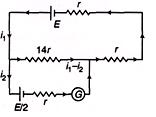Applying loop law in upper loop we have,
E - 14r(i1 - i2) - i1r - i1r = 0 ...(i)
Applying loop law in lower law loop we have,
-E/2 - i2r + (i:- i2)(14r) = 0 ...(ii)
Solving these two equations we get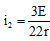Introductory Exercise 20.6

Ques 1: For the given carbon resistor, let the first strip be yellow, second strip be red, third strip be orange and fourth be gold. What is its resistance?
Ans:
Yellow → 4
Red → 2
Orange → 10
Gold → 5
R = (4.2 × 103 + 5%) Ω

Ques 2: The resistance of the given carbon resistor is (2.4 ×106Ω ± 5%)Ω . What is the sequence of colours on the strips provided on resistor?
Ans: 2 → Red
4 → Yellow
106 → Blue
5% → Gold

Offer running on EduRev: Apply code STAYHOME200 to get INR 200 off on our premium plan EduRev Infinity!

132 docs

,

,

,

,

,

,

,

,

,

,

,

,

,

,

,

,

,

,

,

,

,

;